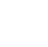Back to Chapter
C++ Setting UpC++ IntroductionNo items found.
C++ BasicsNo items found.
C++ Control StatementsNo items found.
C++ Logical OperatorsNo items found.
C++ Procedural ProgrammingNo items found.
C++ Structural ProgrammingNo items found.
C++ Implementation of OOPSNo items found.
C++ Arrays and VectorsNo items found.
C++ Error HandlingNo items found.
C++ File HandlingNo items found.

# C++ Fibonacci Series

Fibonacci series number in the following sequence 0,1,1,2,3,5,8,13,21,......., and so on. Looking more closely we realize that numbers denoted Fₙ, form a sequence, called the Fibonacci sequence, such that each number is the sum of the two preceding ones, starting from 0 and 1.

Let assume A1 is first number of the sequence A1 = 0, A2=1, A3=A1+A2=1,A4=A3+A2=2 and so on.

Now using what we have learned write a program to print the Fibonacci series for the given number of terms.

``` #include using namespace std; int main() { int n, term1 = 0, term2 = 1, next_Term = 0; cout << "Enter the number of terms: "; cin >> n; cout << "Fibonacci Series: "; for (int i = 1; i <= n; ++i) { // Prints the first two terms. if(i == 1) { cout << t1 << ", "; continue; } if(i == 2) { cout << t2 << ", "; continue; } nextTerm = t1 + t2; term1 = term2; term2 = next_Term; cout << next_Term << ", "; } return 0; } ```
``` Output Enter the number of terms: 5 Fibonacci Series: 0, 1, 1, 2, 3, ```